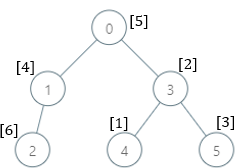# For Solution

There is a family tree rooted at `0` consisting of `n` nodes numbered `0` to `n - 1`. You are given a 0-indexed integer array `parents`, where `parents[i]` is the parent for node `i`. Since node `0` is the root`parents == -1`.

There are `105` genetic values, each represented by an integer in the inclusive range `[1, 105]`. You are given a 0-indexed integer array `nums`, where `nums[i]` is a distinct genetic value for node `i`.

Return an array `ans` of length `n` where `ans[i]` is the smallest genetic value that is missing from the subtree rooted at node `i`.

The subtree rooted at a node `x` contains node `x` and all of its descendant nodes.

## Smallest Missing Genetic Value in Each Subtree leetcode solution```Input: parents = [-1,0,0,2], nums = [1,2,3,4]
Output: [5,1,1,1]
Explanation: The answer for each subtree is calculated as follows:
- 0: The subtree contains nodes [0,1,2,3] with values [1,2,3,4]. 5 is the smallest missing value.
- 1: The subtree contains only node 1 with value 2. 1 is the smallest missing value.
- 2: The subtree contains nodes [2,3] with values [3,4]. 1 is the smallest missing value.
- 3: The subtree contains only node 3 with value 4. 1 is the smallest missing value.
```

Example 2:```Input: parents = [-1,0,1,0,3,3], nums = [5,4,6,2,1,3]
Output: [7,1,1,4,2,1]
Explanation: The answer for each subtree is calculated as follows:
- 0: The subtree contains nodes [0,1,2,3,4,5] with values [5,4,6,2,1,3]. 7 is the smallest missing value.
- 1: The subtree contains nodes [1,2] with values [4,6]. 1 is the smallest missing value.
- 2: The subtree contains only node 2 with value 6. 1 is the smallest missing value.
- 3: The subtree contains nodes [3,4,5] with values [2,1,3]. 4 is the smallest missing value.
- 4: The subtree contains only node 4 with value 1. 2 is the smallest missing value.
- 5: The subtree contains only node 5 with value 3. 1 is the smallest missing value.
```

## Smallest Missing Genetic Value in Each Subtree leetcode solution

```Input: parents = [-1,2,3,0,2,4,1], nums = [2,3,4,5,6,7,8]
Output: [1,1,1,1,1,1,1]
Explanation: The value 1 is missing from all the subtrees.
```

Constraints:

• `n == parents.length == nums.length`
• `2 <= n <= 105`
• `0 <= parents[i] <= n - 1` for `i != 0`
• `parents == -1`
• `parents` represents a valid tree.
• `1 <= nums[i] <= 105`
• Each `nums[i]` is distinct.